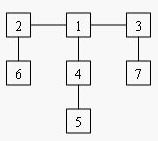# [POJ1655] Balancing Act

## 描述 Description

Consider a tree T with N (1 <= N <= 20,000) nodes numbered 1…N. Deleting any node from the tree yields a forest: a collection of one or more trees. Define the balance of a node to be the size of the largest tree in the forest T created by deleting that node from T.

For example, consider the tree:Deleting node 4 yields two trees whose member nodes are {5} and {1,2,3,6,7}. The larger of these two trees has five nodes, thus the balance of node 4 is five. Deleting node 1 yields a forest of three trees of equal size: {2,6}, {3,7}, and {4,5}. Each of these trees has two nodes, so the balance of node 1 is two.

For each input tree, calculate the node that has the minimum balance. If multiple nodes have equal balance, output the one with the lowest number.

## 输入格式 InputFormat

The first line of input contains a single integer t (1 <= t <= 20), the number of test cases. The first line of each test case contains an integer N (1 <= N <= 20,000), the number of congruence. The next N-1 lines each contains two space-separated node numbers that are the endpoints of an edge in the tree. No edge will be listed twice, and all edges will be listed.

## 输出格式 OutputFormat

For each test case, print a line containing two integers, the number of the node with minimum balance and the balance of that node.

1
7
2 6
1 2
1 4
4 5
3 7
3 1

1 2

POJ 1655

## 代码 Code

``````#include <stdio.h>
#include <iostream>
#include <cstring>
#include <algorithm>
#include <cmath>
using namespace std;
const int inf = 0x7fffffff / 27.11;
const int maxn = 20005;
const int maxm = maxn * 2;
int i, j, t, n, m, l, r, k, z, y, x;
struct edge
{
int to, nx;
} e[maxm];
int head[maxn], used[maxn], son[maxn];
int cnt, num, T, ans, sum;
inline void ins(int u, int v)
{
e[++cnt] = (edge)
{
v, head[u]
}; head[u] = cnt;
}
void dfs(int u, int fa, int tim)
{
int i, t, v;
son[u] = 1;
used[u] = tim;
for (t = 0, i = head[u]; i != 0; i = e[i].nx)
{
v = e[i].to;
if (used[v] != tim && v != fa)
{
dfs(v, u, tim);
son[u] += son[v];
t = max(t, son[v]);
}
}
t = max(t, n - son[u]);
if ((t < sum) || (t == sum && ans > u))
{
ans = u;
sum = t;
}
}
inline void getcenter(int s)
{
ans = 0; sum = inf;
dfs(s, -1, ++num);
printf("%d %d\n", ans, sum);
}
int main()
{
num = 0;
scanf("%d", &T);
while (T--)
{
cnt = 0;
memset(head, 0, sizeof(head));
memset(son, 0, sizeof(son));
scanf("%d", &n);
for (i = 1; i < n; i++)
{
scanf("%d%d", &x, &y);
ins(x, y); ins(y, x);
}
getcenter(1);
}
return 0;
}``````